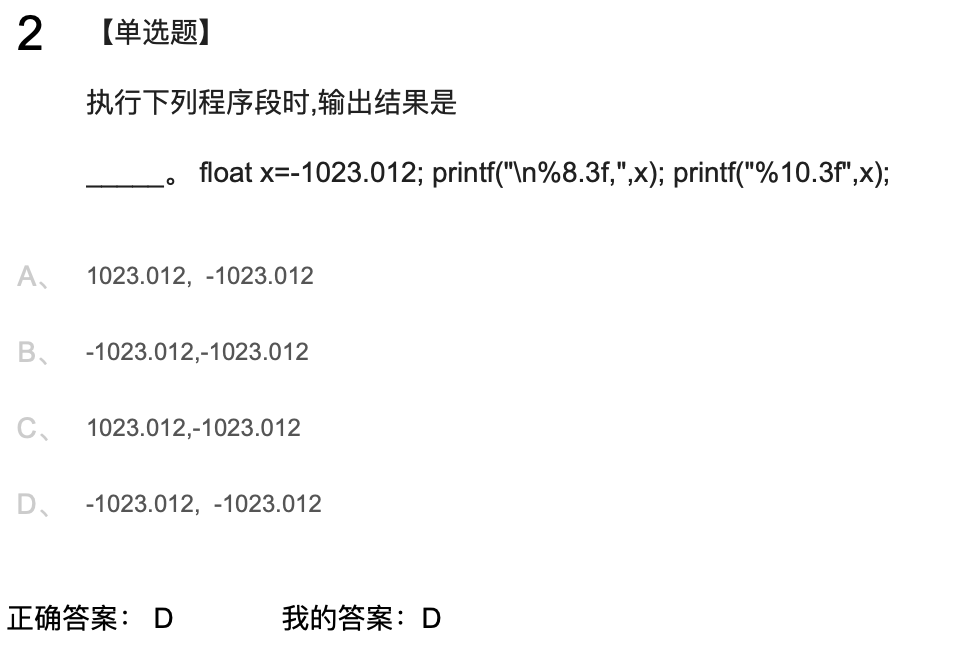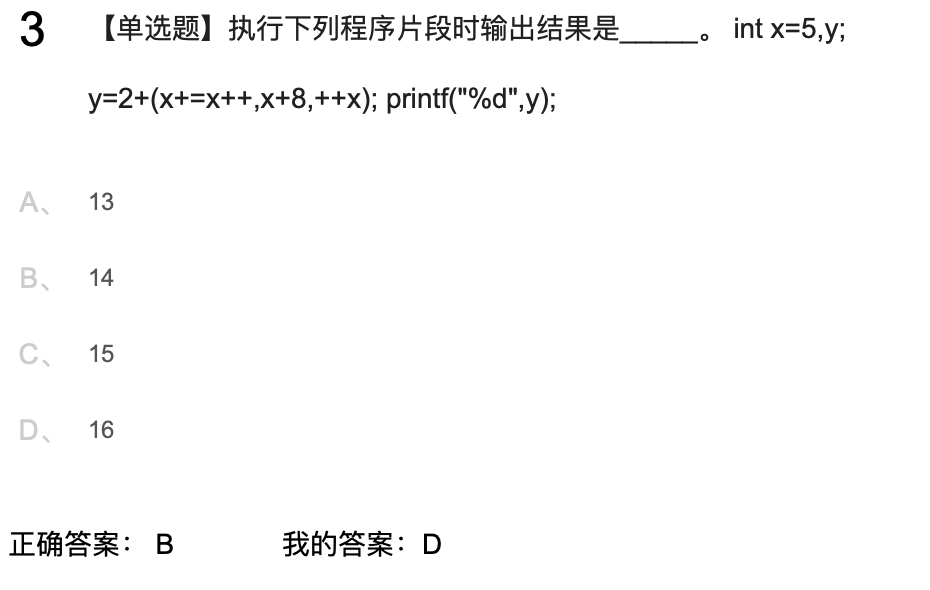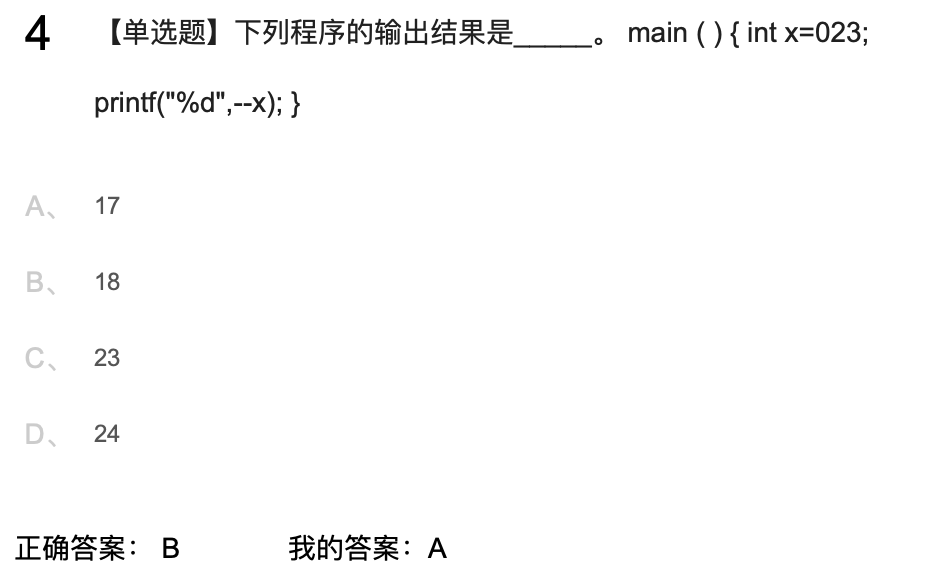2020-03-24 18:23

# c语言基础题，麻烦解答一下谢谢• 点赞
• 写回答
• 关注问题
• 收藏
• 复制链接分享
• 邀请回答

#### 1条回答

• 第二个题目
x=5
x+=x
x=10
x++
x=11
++x
x=12
+2
等于14

最后一个题目
x=023（八进制）=2x8+3=19
--x
18

点赞 评论 复制链接分享